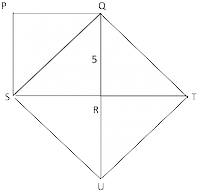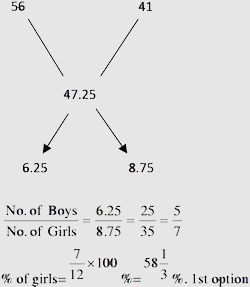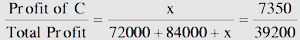# Sample Aptitude Questions of Global-Logic

DIRECTIONS for the question 1: Solve the following question and mark the best possible option.
1. I. 10x2 + 15x + 5 = 0
II. 4y2 + 17y + 15 = 0
1. x > y
2. x < y
3. x ≥ y
4. Relationship between x and y cannot be established.
5. x ≤ y
I. 10x2 +15x + 5=0 ⇒x= -1, -1/2
II.  4y2 +17y+15=0 ⇒ y = -3, -5/4
For x = -1 ⇒-1 > -3  and -1 > -5/4
For x = -1/2 ⇒ -1/2 > -3 and -1/2 > -5/4
So we get x > y. Hence A option
2. In the figure given below, PQRS is a square with side 5cms. Triangles QST and UTS are congruent. R is midpoint of ST. What is the area of quadrilateral QSUT in cm2 ?.1. 25 cm2
2. 36 cm2
3. 55 cm2
4. 50 cm2
5. 100 cm2Diagonal QS of square PQRS = √2 * a = √2 * 5 cm
Since ΔQST ΔUTS
OS = UT = 5√2, also QT = US
SR = RT = 5cm as R is the mid point given
QR = 5cm. and also ∠RQ = 90° as PQRS is a square.
So QRT=90° (By linear Pair).
In Rt. ΔQRT by Pythagoras
QT2 = QR2+RT2
QT = 5√2 = US,
Similarly in Rt. ΔSRU, RU=5cm,
Now QSUT is a quadrilateral with all sides equal & diagonals ⊥ and equal, so QSUT is a square.
Area of QSUT = 5√2 * 5√2 = 50 cm2
Hence, option D.
1. The average weight of boys in a class is 56 kg and the average weight of girls in the same class is 41 kg. If the average weight of the entire class is 47.25 kg, then find that the number of girls constitutes what percent of the total number of students in the class?
1. 5813
2. 6323
3. 5623
4. 5413
5. 60
Using the rule of alligation,DIRECTIONS for the question 4: In the following series, one of the terms given is wrong. Find that term and mark that as your answer.
2. 13 16 24 39 73 98
1. 16
2. 73
3. 39
4. 96
5. 24
The first set of differences are
+3,+8,+15,+24,+35,+48
And again the differences are
+5,+7,+9,+11,+13…….which are in AP
So following the logic we get that 73 is wrong. So Ans is B.
3. Equal sums of money were invested in scheme A and scheme B for two years. Scheme A offers simple interest and scheme B offers compound interest (compounded annually) and the rate of interest (p.c.p.a) for both the schemes are same. The interest accrued from Scheme A after two years is Rs. 2560/- and from scheme B is Rs. 2688/-. Had the rate of interest (p.c.p.a) of scheme A been 6% more, what would have been the interest accrued from Scheme A after two years?
1. Rs. 3972/-
2. Rs. 4124/-
3. Rs. 4266/-
4. Rs. 4096/-
5. Rs. 4154/-
The SI for 2 years=Rs.2560/-
Since simple interest for each year is same.
SI per annum is Rs.1280
CI for 2 years is Rs.2688/-
Since the rate of interest is same
So CI for 1st year is Rs.1280
CI for 2nd year is (Rs. 1280 + Rs.128)
Hence Rate of interest per annum is = 128/1280×100% = 10%
If the rate of interest is increased by 6% then SI for 2 years = 16%/10%×2560 =  Rs. 16×256 = Rs.4096.
4. A & B started a business together by investing Rs. 36000/- and Rs. 42000/- respectively. Both of them invested for one year whereas after 6 months from the start C joined them by investing a certain amount. If they earned an annual profit of Rs. 39,200/- out of which C’s share is Rs. 7350/-. What is the investment of C?
1. Rs. 24000/-
2. Rs. 36000/-
3. Rs. 27000/-
4. Rs. 33000/-
5. Rs. 30000/-
Let the investment of C=Rs x
Ratio of profits of A &B &C
=36000×12 : 42000×12 : x×6
=72000:84000: xSolving, x = 36000. B option.
5. What are the marks obtained by Harish in Maths ?
I. Average marks obtained by Harish in Maths and economies are 83.
II. Average marks obtained by Harish in Maths and statistics are 92.
1. The data in statement I alone are sufficient to answer the question, while the data in statement II alone not sufficient to answer the question.
2. The data in both the statements I & II together are necessary to answer the question.
3. The data either in statement I alone or in statement II are sufficient to answer the question.
4. The data in both the statements I & II together are insufficient to answer the question.
5. The data in statement II alone are sufficient to answer the question, while the data in statement I alone are not sufficient to answer the question.
We cannot find the marks in maths  alone even by using both the statements together as average marks will not tell us exact marks in Maths.
Data is insufficient.  Hence D option.
6. By what percent is Rajesh’s salary more/less than Mangesh’s ?
I. Salary of Rajesh is Rs. 12000/-.
II. Salary of Rajesh and Mangesh together is Rs 28000/-
1. The data in statement I alone are sufficient to answer the question, while the data in statement II alone not sufficient to answer the question.
2. The data in both the statements I & II together are necessary to answer the question.
3. The data either in statement I alone or in statement II are sufficient to answer the question.
4. The data in both the statements I & II together are insufficient to answer the question.
5. The data in statement II alone are sufficient to answer the question, while the data in statement I alone are not sufficient to answer the question.
By using both the statements together we can find the salary of Mangesh . Hence we can find the percentage change between the salaries of Rajesh and Mangesh. B option.
7. What is the strength of MBA institute where students study only HR, Marketing and Finance ?
I. Number of students studying HR, Marketing and Financial are in the ratio of 2 : 3: 5 respectively.
II. Number of students studying Marketing is more than those studying HR by 800.
1. The data in statement I alone are sufficient to answer the question, while the data in statement II alone are not sufficient to answer the question.
2. The data either in statement I alone or in statement II alone are sufficient to answer the question.
3. The data in both the statements I & II together are necessary to answer the question.
4. The data in statement II alone are sufficient to answer the question, while the data in statement I alone not sufficient to answer the question.
5. The data in both the statements I & II together are insufficient to answer the question.
Using both statements together , Let no. of students of HR, marketing and Finance be 2x,3x,5x and also 3x-2x=800
x=800
So total students =2x+3x+5x=10×800=8000. Hence C.
8. A shopkeeper purchased 96 identical shirts @ Rs. 220/- each. He spent Rs. 3800/- on transport and packing and fixed a marked price of Rs. 450/- each shirt. However, he decided to give discount of 20% on the marked price of each shirt. If he could sell all the shirts, what is the approximate percent profit earned by him?
1. 44
2. 39
3. 33
4. 31
5. 30